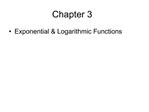DownloadDownload PresentationExponential Logarithmic Functions

# Exponential Logarithmic Functions

Télécharger la présentation## Exponential Logarithmic Functions

- - - - - - - - - - - - - - - - - - - - - - - - - - - E N D - - - - - - - - - - - - - - - - - - - - - - - - - - -
##### Presentation Transcript

1. Chapter 3 Exponential & Logarithmic Functions

2. 3.1 Exponential Functions Objectives Evaluate exponential functions. Graph exponential functions. Evaluate functions with base e. Use compound interest formulas.

3. Definition of exponential function How is this different from functions that we worked with previously? Some DID have exponents, but NOW, the variable is found in the exponent. (example is NOT an exponential function)

4. Common log When the word �log� appears with no base indicated, it is assumed to be base 10. Using calculators, �log� button refers to base 10. log(1000) means to what EXPONENT do you raise 10 to get 1000? 3 log(10) = -1 (10 raised to the -1 power=1/10)

5. Graph of an exponential function Graph As x values increase, f(x) grows RAPIDLY As x values become negative, with the magnitude getting larger, f(x) gets closer & closer to zero, but with NEVER = 0. f(x) NEVER negative

6. Other characteristics of ______ The y-intercept is the point (0,1) (a non-zero base raised to a zero exponent = 1) If the base b lies between 0 & 1, the graph extends UP as you go left of zero, and gets VERY close to zero as you go right. Transformations of the exponential function are treated as transformation of polynomials (follow order of operations, x�s do the opposite of what you think)

7. Graph ____________ Subtract 3 from x-values (move 3 units left) Subtract 4 from y-values (move 4 units down) Note: Point (0,1) has now been moved to (-3,-3)

8. Applications of exponential functions Exponential growth (compound interest!) Exponential decay (decomposition of radioactive substances)

9. 3.2 Logarithmic Functions Objectives Change from logarithmic to exponential form. Change from exponential to logarithmic form. Evaluate logarithms. Use basic logarithmic properties. Graph logarithmic functions. Find the domain of a logarithmic function. Use common logarithms. Use natural logarithms.

10. logarithmic and exponential equations can be interchanged

11. Rewrite the following exponential expression as a logarithmic one.